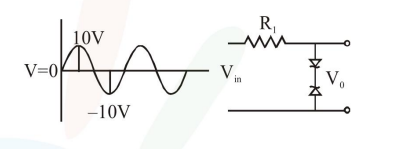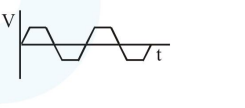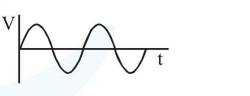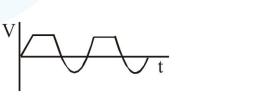# Solve this following

Question:

Take the breakdown voltage of the zener diode used in the given circuit as 6V. For the input voltage shown in figure below, the time variation of the output voltage is : (Graphs drawn are schematic and not to scale)1.2.3.4.Correct Option: 2,

Solution:

As there are two zener diodes in reverse polarity so if one is in forward bias the other will be in reverse bias and above $6 \mathrm{~V}$ the reverse bias will too be in conduction mode. Therefore when voltage is more than $6 \mathrm{~V}$ the output will be constant. And when it is less than $6 \mathrm{~V}$ it will follow the input voltage so correct answer is two.